Bioinformatic Analyses of 2009-2010 Pandemic H1N1 Influenza A Hemagglutinin Subsets | OMICS International
Journal of Medical Microbiology & Diagnosis

Like us on:

# Bioinformatic Analyses of 2009-2010 Pandemic H1N1 Influenza A Hemagglutinin Subsets

William A Thompson1 and Joel K Weltman2*

1Division of Applied Mathematics and Center for Computational Molecular Biology, Brown University, Providence, RI 02912, USA

2Department of Medicine, Alpert/Brown University School of Medicine, Providence, RI 02912, USA

*Corresponding Author:
Joel K Weltman
Department of Medicine
Alpert/Brown University School of Medicine
Providence, RI 02912, USA
E-mail: [email protected]

Received Date: April 11, 2012; Accepted Date: May 09, 2012; Published Date: May 16, 2012

Citation: Thompson WA, Weltman JK (2012) Bioinformatic Analyses of 2009-2010 Pandemic H1N1 Influenza A Hemagglutinin Subsets. J Med Microb Diagn 1:110. doi: 10.4172/2161-0703.1000110

Copyright: © 2012 Thompson WA, et al. This is an open-access article distributed under the terms of the Creative Commons Attribution License, which permits unrestricted use, distribution, and reproduction in any medium, provided the original author and source are credited.

Visit for more related articles at Journal of Medical Microbiology & Diagnosis

#### Abstract

We report here an analysis of mutations in the 2009-2010 pandemic H1N1 influenza Ahemagglutinin gene (HA) based upon information entropy (H), Mutual Information (MI) and geography. The purpose of this study is to determine whether the processes that dominated the evolution of the pandemic virus were either non-random or random. The complete pandemic dataset was bisected into two subsets according to the nucleotide occupying the position of maximum H. The resulting subsets were almost disjoint with respect to overall H distribution, with correlation of H less than that of randomly formed subsets. It was further found that MI between the two nucleotide positions of greatest H was associated with an asymmetric, non-random distribution of mutant counts. The cumulative distributions of pandemic HA sequences from 23 geographic locations world-wide were represented by a system of equations that yielded sequence distributions that were in concordance with available epidemiological/clinical data. It is concluded that the non-random distributions and correlations observed for the HA gene in this research reflect non-random, deterministic biological forces that influenced the evolutionary trajectory of the 2009 – 2010 H1N1 pandemic influenza virus.

#### Introduction

Influenza remains a global threat and a significant public health problem . Insight into the process of influenza viral variation can help inform public health policy, antiviral drug development and protective vaccine design. We report here a bioinformatic analysis of subsets of the Hemagglutinin (HA) gene of the 2009-2010 pandemic H1N1 influenza virus. Subset sorting was based upon the HA nucleotide position of maximum information entropy (H). The sorting of HA gene mutants reported here is consistent with the non-random organization of intergenic mutual information in the pandemic virus previously reported . The results of this research suggest that this intra- and intergenic organization may provide targets for analyzing, and perhaps, manipulating the evolutionary trajectory of the influenza virus.

#### Materials and Methods

The entire dataset of 3460 hemagglutinin (HA) sequences from the 2009-2010 H1N1 pandemic was downloaded from the NCBI Influenza Virus Resource Database  on November 26, 2010. The dataset was comprised of H1N1 influenza A HA sequences obtained from human patients world-wide between March 30, 2009 and April 4, 2010. Sequences either with nucleotides identified as other than A, C, G or U/T or which terminated before position 1701 were excluded. A total of 3382 (97.75%) of these HA sequences were of sufficient length and quality to be used for this analysis. The nucleotides of the influenza virus HA gene are referred to in this report with numbering of the 1701 nucleotide positions relative to the 5'-terminus of the mRNA.

Computations were performed with Python 2.6.4  with SciPy 0.7.1 , Numpy 1.3.0 and matplotlib . Information entropy (H) was computed according to Shannon . Mutual information (MI) was computed according to Equation 2.28 in Cover and Thomas . Z score probabilities are reported as two-tailed. Standard deviations for z scores were each obtained from 1000 pseudorandom trials.

In order to facilitate comparison of the kinetics of acquisition of HA subset sequences, each cumulative sequence count (Y) was approximated as a continuous integral function (Equation 1):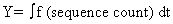(1)

A logistic-type function  was fit to Y by regression to cumulative, sequence counts obtained for the HA sequence subsets for the entire pandemic period: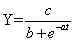(2)

Where t represents time (t), a and b are non-linear parameters and c is a linear parameter. Values of the parameters a, b and c were determined for each subset by regression to the observed cumulative sequence counts, as a continuous, integral over t (time).

The parameter values were used to obtain numerical values for the first (Y'), second (Y'') and third (Y''') derivatives of Y with respect to time according to Equations 3 – 5: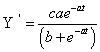(3)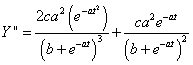(4)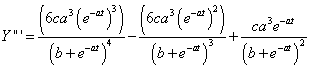(5)

Differentiation of Equation (2) with respect to time and fitting of values to parameters a, b, and c to Equation (2) by regression was performed with Maple 15.01 (Maplesoft, a division of Waterloo Maple, Inc.).

Subsets were formed from the complete dataset of HA sequences depending upon the nucleotide occupying the position of maximum entropy. Subsets were further classified according to geographic origin for locations from which at least 20 HA sequences had been collected during the pandemic.

#### Results

The distribution of information entropy (H) in the HA gene sequences of the complete dataset is shown in Figure 1. The mean and median H values are 0.01409 and 0.0039, respectively with standard deviation = 0.0417. The H distribution in Figure 1 is highly asymmetric (skew = 11.0517, z score = 45.7727, p<2.22E-16) and peaked (kurtosis = 170.9245).There are 11 nucleotide positions with H greater than the mean plus 4 standard deviations. This subset of statistical outliers consisted of the following nucleotide positions (z scores and p values in parentheses): position 4 (5.8456, 5.0487e-09), position 145 (8.2279, 1.9052e-16), position 340 (5.3584, 8.3965e-08), position 658 (20.5529, <2.22e-16), position 717 (7.6279, 2.3864e-14), position 852 (4.9299, 8.2270e-07), position 930 (6.8301, 8.4828e-12), position 1012 (7.1721, 7.3832e-13), position 1171 (13.7875, <2.22e-16), position 1281 (11.5394, <2.22e-16) and position 1408 (14.6859, <2.22e-16).

Figure 1: Information Entropy (H) Distribution in the Pandemic 2009-2010 H1N1 HA Gene. The red dashed line represents the mean plus four standard deviations. H is expressed in bits.

The position of maximum entropy (position 658) was used as a basis for sorting the complete set of HA sequences from the pandemic into two subsets, according to the nucleotide occupying that position (either A658 or U658). The A658 subset was comprised of 2406 sequences while the U658 subset was comprised of 976 sequences (z score = 23.6085, p < 2.22e-16). There was no significant correlation (Pearson r =0.1879) between the distributions of entropy in the A658 and U658 subsets (Figure 2). In contrast, the correlation between entropy values of randomly formed subsets of the same sequences was highly significant (Pearson r = 0.9633, p < 2.22e-16).The six extreme outliers along the A658 subset axis in Figure 2 were identified as (H values in parentheses): position 340 (0.2947), position 717 (0.4255), position 852 (0.2771), position 1012 (0.3575), position 1171 (0.7193) and position 1281 (0.6059). The four extreme outliers along the U658 subset axis in Figure 2 were identified as: position 4 (0.5988), position 145 (0.7195), position 930 (0.6363) and position 1408 (0.9963). These outlier nucleotide positions are the same as those identified as statistical outliers in Figure 1.

Figure 2: Relation Between Information Entropy (H) Distributions in HA (A658) and HA(U658) Subsets. Observed H values (black circles) for nucleotide positions of subset A658 sequences are on the abscissa and for nucleotide positions of subset U658 sequences are on ordinate. H values for nucleotide positions of random subsets of the sequences are shown as red circles. The dotted red line is a reference straight line with slope = 1.0 and intercept = 0.0.

The mutual information (MI) distribution of the HA gene sequence, with nucleotide position 658 as the reference position, is shown in Figure 3. The MI of position 1408 exceeds the mean MI plus four standard deviations. The counts of A, C, G and T(U) nucleotides at position 1408 of the A658 subset of sequences were determined to be 0, 7, 0 and 2396.The corresponding counts for the U658 subset of sequences were 0, 523, 0 and 453. Thus, there were 74.7 times the number of sequences with C at position 1408 in the U658 subset (523 sequences) than were in theA658 subset (7 sequences) yielding for [7,5,23] a z score = 22.8854 (p < 2.22e-16).

Figure 3: Distribution of HA Nucleotide Position 658 Mutual Information in the HA Gene of the 2009-2010 Pandemic H1N1 Influenza Virus.The dashed red line represents the mean plus 4 standard deviations.

The time course for the acquisition of HA sequences in the A658 and U658 subsets of the entire HA dataset for the pandemic is shown in Figure 4. The observed cumulative sequence counts are given in Figure 4 (upper). The observed time course for the A658 subset is different from that of the U658 subset, with A658 emerging as dominant on day 127 of the pandemic. The total cumulative sequence counts (A658= 2406, U658= 976) that were obtained in these time series differed significantly from each other (z score =22.4780, p<2.22e-16). The time to reach ½ of the of the total summed count, ie, time to half-maximum, was 178 days for the A658 subset and 58 days for the U658 subset (zs core = 7.7125, p=1.2338e-14). The Spearman r for the correlation between the observed cumulative curve of sequence counts and Y values calculated

Figure 4: HA Subset Global Sequence Acquisition Kinetics. Results for A658 subset sequences in red and results for U658 subset sequences are in blue.

according to Equation (2) was r = 0.9999 (p < 2.22e-16) for the A658 subset and r = 0.9995 (p < 2.22e-16) for the U658 subset of sequences. Numerical values of parameters a, b and c determined from Equation 2 by regression were a = 0.0184, b = 0.0419and c = 107.8571 for the A658 HA sequence subset and a = 0.0411, b = 0.0872 and c = 83.2110 for the U658 subset of HA sequences. The numerical values obtained with these parameters for the derivatives Y', Y'' and Y''' for the A658 subset clearly differed from those obtained for the U658 subset (see Supplementary Figure 1). Values for Y', shown in Figure 4 (lower), are approximations to the daily raw sequence counts with smoothing of the spikes.

Twenty-three (23) geographic locations were identified world-wide at which at least 20 pandemic HA nucleotide sequences had been deposited in the NCBI influenza database. The sequence counts for A658 and U658 subsets from these 23 locations are given in Table 1. Overall, there was no correlation between the sequence counts in the A658 subsets and in the U658 subsets (Pearson r = 0.2739, p=0.2060; Spearman r=0.0803, p= 0.7157). At six of the locations (Argentina, Australia, Chile, Egypt, Madrid and Malaysia) only members of the A658 sequence subset were reported, i.e, the U658 subset was empty at each of these geographic locations. For all instances of the reported viral sequences (Supplementary Table 1), there was high correlation between the observed cumulative sequence counts and the values of Y calculated from Equation 2, with a minimum correlation (Pearson r = 0.9495, p < 2.22e- 16) for California and maximum (Pearson r = 0.9991, p < 2.22e-16) for Thailand and for Wisconsin. See the Supplementary Figures 2-4 for graphs of Y, Y', Y'' and Y''' for the HA (A658) and HA (U658) subsets at all 23 locations.

Geographic Location Sequence Count
A658 Subset
Sequence Count
U658 Subset
Zscore, p (z)
Afghanistan 9 20 1.9633, 0.0496
Argentina 32 0 5.6900, 1.2706e-08
Australia 57 0 7.9120, 2.5335e-15
Boston 39 27 1.4825, 0.1382
California 124 34 7.1839, 6.7749e-13
Chile 49 0 6.9011, 5.1618e-12
Egypt 37 0 6.0651, 1.3190e-09
Finland 117 10 9.75362, 1.7805e-22
Houston 4 26 4.0223, 5.7621e-05
Kuwait 7 17 2.0522, 0.0401
Malaysia 42 0 6.5512, 5.7094e-11
Managua 34 96 5.5279, 3.2411e-08
Mexico 7 72 7.2833, 3.2583e-13
Nagasaki 67 12 6.1698, 6.8396e-10
NewYork 346 47 15.0578, 3.0671e-51
Ontario 26 12 2.2398, 0.0251
SanDiego 54 13 5.0878, 3.6233e-07
Singapore 114 9 9.4229, 4.3872e-21
Texas 155 58 6.6027, 4.0367e-11
Thailand 27 36 1.1378, 0.2552
Wisconsin 137 190 2.8408, 0.0045

Table 1: Geographic Distribution of A658 and U658 Pandemic Influenza Hemagglutinin Subsets. Sequence count colors: red (A658>U658); blue (A658<U658); black (A658≅U658).

From one of the geographic locations in the study (Wisconsin, USA), published epidemiological and clinical data were available in the literature . The rate of hospitalization for influenza illness (Figure 5) is consistent with Y' values calculated from Equation 3. Note the wavelike distribution of HA sequences in Figure 5, predicted from Equation 3 and observed for the hospitalization rate. It was reported by the Department of Health of the State of Wisconsin that influenza illness in the second wave, (HA (A658) was more severe than in the first wave (HA (U658)).

Figure 5: HA Subset Local Sequence Acquisition Kineticsforthe state of Wisconsin, USA. Results for A658 subset sequences are in red and results for U658 subset sequences are in blue. The hospitalization rate per 100, 000 population s (dashed black, vertical lines) wasadapted from Truelove, Chitnis, Heffernan et al .

#### Discussion

The evolutionary trajectory of the influenza A virus reflects interactions among viral genetics, host genetics and environmental factors . The A658U transversion in the HA gene of the pandemic virus changes the ACA codon to UCA, thereby producing the T203S mutation in the HA protein. (Mutation T203S is designated T206S in H3 numbering; see Garten et al. ). Mutation HA T203S has been shown to have played an important role in the global and local organization of 2009-2010 pandemic influenza viral strains into clusters and clades [13-17]. The molecular and cellular mechanisms of theT203S effects on influenza viral organization have not yet been determined. Despite its proximity to a surface epitope, no effect of the HA T203S mutation on the antigenicity of the virus has been demonstrated [12,13].

The primary question addressed in this research is whether the propagation of influenza virus mutations during the 2009-2010 H1N1 pandemic was random or non-random. The sorting of HA sequences from the pandemic, based upon the nucleotide identity at the position of maximum entropy (Figure 1) produced two subsets with correlation of information entropy clearly less than that of randomly formed subsets (Figure 2). Furthermore, the mutual information (Figure 3) between the two HA nucleotide positions of greatest entropy (positions 658 and 1408) represented an asymmetric distribution of mutants with probability smaller even than the epsilon number (2.22e-16) of the computer used (see Results). These results suggest that the point-mutational evolutionary trajectory of the pandemic H1N1 was highly non-random. Three of the ten HA mutations that displayed almost-disjoint distributions of mutations (Figure 2) were sites of synonymous mutations (U852C, A1281G and U1408C) thereby suggesting interactions of covarying mutations at the nucleotide level. These results are consistent with the non-random intergenic interactions in the pandemic influenza virus recently reported in which seven of the nine mutating, interacting nucleotide positions were sites of synonymous mutations . It has been demonstrated in bacteria, that rates of protein synthesis are regulated at the ribosomal level by synonymous mutation .

The viral subsets were analyzed by a set of Ordinary Differential Equation(S) (ODEs) (Equations 3-5) derived from an integral equation (Equation 2). Application of the equations to the viral sequence counts results in a curve-fitting step (Figure 4 top) and a curve-smoothing step (Figure 4 bottom). A biomedical significance of these equations is suggested by the observed concordance between the predicted time course of their derivatives and the wave-like epidemiological and clinical descriptions of the pandemic (Figure 5). Wave-like behavior is a characteristic that has been reported for previous, more deadly influenza pandemics .

The equations used in this study are continuous and differentiable, yielding algebraic solutions for the differentiation operation. See Supplementary Figures 1-4 for graphs in which Equations 2-5 were applied to every subset of HA sequences considered here.

is proposed that the non-random correlations of mutations reported here reflect deterministic, intragenic regulatory processes that occurred within the HA gene of the 2009 – 2010 H1N1 pandemic influenza virus and that describing and understanding these processes can be helpful in tracking and managing future influenza epidemics.

#### Acknowledgements

The authors thank the Brown University Center for Computing and Visualization for providing computer facilities and ancillary support for this research. The authors also thank the Brown University Center for Computational Molecular Biology and the Department of Medicine of the Alpert/Brown University School of Medicine for research support. The authors thank Andy Martwick of Portland State University and the Intel Corporation for helpful discussions.

#### References

Select your language of interest to view the total content in your interested language

### Article Usage

• Total views: 12523
• [From(publication date):
June-2012 - Jan 19, 2020]
• Breakdown by view type
• HTML page views : 8724Can't read the image? click here to refresh